This book is archived and will be removed July 6, 2022. Please use the updated version.

Quantum Mechanics

# 52 The Schrӧdinger Equation

### Learning Objectives

By the end of this section, you will be able to:

• Describe the role Schrӧdinger’s equation plays in quantum mechanics
• Explain the difference between time-dependent and -independent Schrӧdinger’s equations
• Interpret the solutions of Schrӧdinger’s equation

In the preceding two sections, we described how to use a quantum mechanical wave function and discussed Heisenberg’s uncertainty principle. In this section, we present a complete and formal theory of quantum mechanics that can be used to make predictions. In developing this theory, it is helpful to review the wave theory of light. For a light wave, the electric field E(x,t) obeys the relation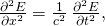where c is the speed of light and the symbol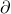represents a partial derivative. (Recall from Oscillations that a partial derivative is closely related to an ordinary derivative, but involves functions of more than one variable. When taking the partial derivative of a function by a certain variable, all other variables are held constant.) A light wave consists of a very large number of photons, so the quantity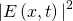can interpreted as a probability density of finding a single photon at a particular point in space (for example, on a viewing screen).

There are many solutions to this equation. One solution of particular importance is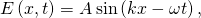where A is the amplitude of the electric field, k is the wave number, and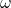is the angular frequency. Combing this equation with (Figure) gives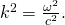According to de Broglie’s equations, we have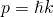and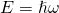. Substituting these equations in (Figure) gives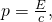or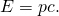Therefore, according to Einstein’s general energy-momentum equation ((Figure)), (Figure) describes a particle with a zero rest mass. This is consistent with our knowledge of a photon.

This process can be reversed. We can begin with the energy-momentum equation of a particle and then ask what wave equation corresponds to it. The energy-momentum equation of a nonrelativistic particle in one dimension is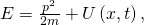where p is the momentum, m is the mass, and U is the potential energy of the particle. The wave equation that goes with it turns out to be a key equation in quantum mechanics, called Schrӧdinger’s time-dependent equation.

The Schrӧdinger Time-Dependent Equation

The equation describing the energy and momentum of a wave function is known as the Schrӧdinger equation: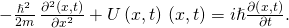As described in Potential Energy and Conservation of Energy, the force on the particle described by this equation is given by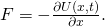This equation plays a role in quantum mechanics similar to Newton’s second law in classical mechanics. Once the potential energy of a particle is specified—or, equivalently, once the force on the particle is specified—we can solve this differential equation for the wave function. The solution to Newton’s second law equation (also a differential equation) in one dimension is a function x(t) that specifies where an object is at any time t. The solution to Schrӧdinger’s time-dependent equation provides a tool—the wave function—that can be used to determine where the particle is likely to be. This equation can be also written in two or three dimensions. Solving Schrӧdinger’s time-dependent equation often requires the aid of a computer.

Consider the special case of a free particle. A free particle experiences no force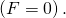Based on (Figure), this requires only that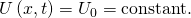For simplicity, we set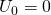. Schrӧdinger’s equation then reduces to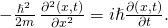A valid solution to this equation is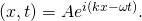Not surprisingly, this solution contains an imaginary number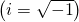because the differential equation itself contains an imaginary number. As stressed before, however, quantum-mechanical predictions depend only on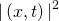, which yields completely real values. Notice that the real plane-wave solutions,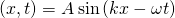and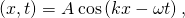do not obey Schrödinger’s equation. The temptation to think that a wave function can be seen, touched, and felt in nature is eliminated by the appearance of an imaginary number. In Schrӧdinger’s theory of quantum mechanics, the wave function is merely a tool for calculating things.

If the potential energy function (U) does not depend on time, it is possible to show that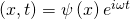satisfies Schrӧdinger’s time-dependent equation, where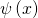is a time-independent function andis a space-independent function. In other words, the wave function is separable into two parts: a space-only part and a time-only part. The factoris sometimes referred to as a time-modulation factor since it modifies the space-only function. According to de Broglie, the energy of a matter wave is given by, where E is its total energy. Thus, the above equation can also be written as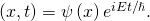Any linear combination of such states (mixed state of energy or momentum) is also valid solution to this equation. Such states can, for example, describe a localized particle (see (Figure))

Check Your Understanding A particle with mass m is moving along the x-axis in a potential given by the potential energy function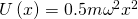. Compute the product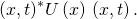Express your answer in terms of the time-independent wave function,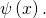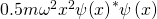Combining (Figure) and (Figure), Schrödinger’s time-dependent equation reduces to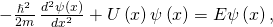where E is the total energy of the particle (a real number). This equation is called Schrӧdinger’s time-independent equation. Notice that we use “big psi”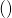for the time-dependent wave function and “little psi”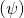for the time-independent wave function. The wave-function solution to this equation must be multiplied by the time-modulation factor to obtain the time-dependent wave function.

In the next sections, we solve Schrӧdinger’s time-independent equation for three cases: a quantum particle in a box, a simple harmonic oscillator, and a quantum barrier. These cases provide important lessons that can be used to solve more complicated systems. The time-independent wave functionsolutions must satisfy three conditions:

•must be a continuous function.
• The first derivative ofwith respect to space,, must be continuous, unless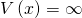.
•must not diverge (“blow up”) at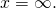The first condition avoids sudden jumps or gaps in the wave function. The second condition requires the wave function to be smooth at all points, except in special cases. (In a more advanced course on quantum mechanics, for example, potential spikes of infinite depth and height are used to model solids). The third condition requires the wave function be normalizable. This third condition follows from Born’s interpretation of quantum mechanics. It ensures that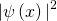is a finite number so we can use it to calculate probabilities.

Check Your Understanding Which of the following wave functions is a valid wave-function solution for Schrӧdinger’s equation?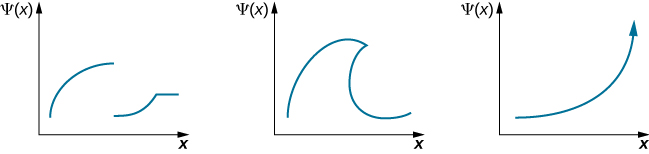None. The first function has a discontinuity; the second function is double-valued; and the third function diverges so is not normalizable.

### Summary

• The Schrӧdinger equation is the fundamental equation of wave quantum mechanics. It allows us to make predictions about wave functions.
• When a particle moves in a time-independent potential, a solution of the time-dependent Schrӧdinger equation is a product of a time-independent wave function and a time-modulation factor.
• The Schrӧdinger equation can be applied to many physical situations.

### Conceptual Questions

What is the difference between a wave function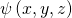and a wave function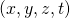for the same particle?

If a quantum particle is in a stationary state, does it mean that it does not move?

No, it means that predictions about the particle (expressed in terms of probabilities) are time-independent.

Explain the difference between time-dependent and -independent Schrӧdinger’s equations.

Suppose a wave function is discontinuous at some point. Can this function represent a quantum state of some physical particle? Why? Why not?

No, because the probability of the particle existing in a narrow (infinitesimally small) interval at the discontinuity is undefined.

### Problems

Combine (Figure) and (Figure) to showCarrying out the derivatives yieldsShow that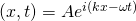is a valid solution to Schrӧdinger’s time-dependent equation.

Show thatand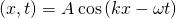do not obey Schrӧdinger’s time-dependent equation.

Carrying out the derivatives (as above) for the sine function gives a cosine on the right side the equation, so the equality fails. The same occurs for the cosine solution.

Show that when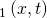and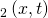are solutions to the time-dependent Schrӧdinger equation and A,B are numbers, then a function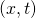that is a superposition of these functions is also a solution: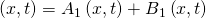.

A particle with mass m is described by the following wave function: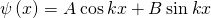, where A, B, and k are constants. Assuming that the particle is free, show that this function is the solution of the stationary Schrӧdinger equation for this particle and find the energy that the particle has in this state.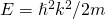Find the expectation value of the kinetic energy for the particle in the state,. What conclusion can you draw from your solution?

Find the expectation value of the square of the momentum squared for the particle in the state,. What conclusion can you draw from your solution?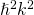; The particle has definite momentum and therefore definite momentum squared.

A free proton has a wave function given by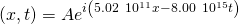.

The coefficient of x is inverse meters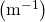and the coefficient on t is inverse seconds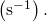Find its momentum and energy.

### Glossary

Schrӧdinger’s time-dependent equation
equation in space and time that allows us to determine wave functions of a quantum particle
Schrӧdinger’s time-independent equation
equation in space that allows us to determine wave functions of a quantum particle; this wave function must be multiplied by a time-modulation factor to obtain the time-dependent wave function
time-modulation factor
factorthat multiplies the time-independent wave function when the potential energy of the particle is time independent